Get instant live expert help with Excel or Google Sheets“My Excelchat expert helped me in less than 20 minutes, saving me what would have been 5 hours of work!”

#### Post your problem and you’ll get Expert help in seconds.

Your message must be at least 40 characters
Our professional Expert are available now. Your privacy is guaranteed.

# Highlight cells that equal

Excel provides an easy way of highlighting cells based on a given condition or criteria by using conditional formatting.  This step by step tutorial will assist all levels of Excel users in highlighting cells that equal to a certain value.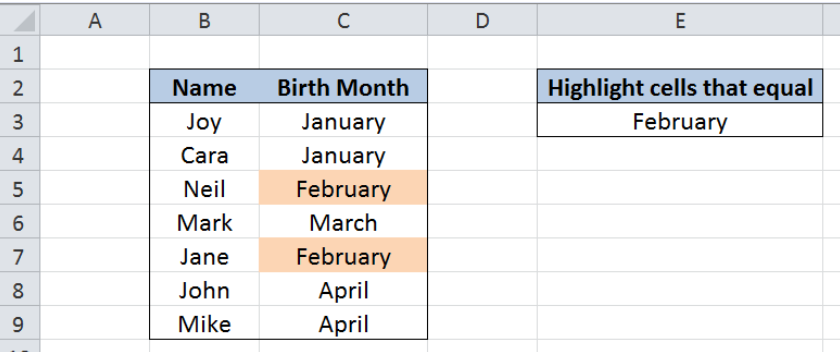Figure 1.  Final result: Highlight cells that equal to a value

## Setting up the Data

Our table consists of two columns:  Name (column B) and Birth Month (column C).  In cell E3, we enter our criteria “February”.  We want to use conditional formatting to highlight the cells that equal to the value in cell E3, or “February”.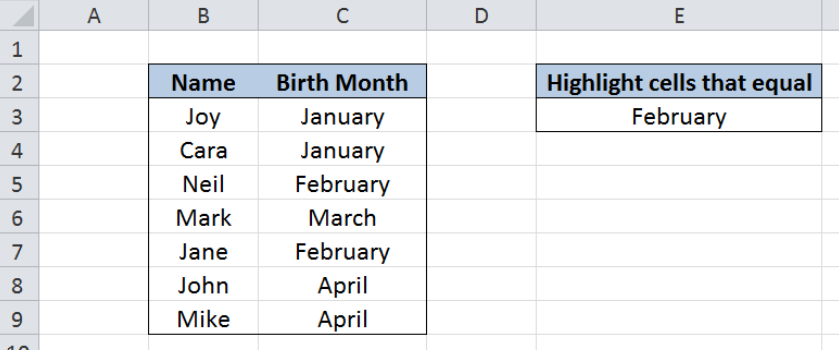Figure 2.  Sample data to highlight cells that equal a value

## Highlight cells that equal to cell E3

We want to highlight the cells in column C that equal to the value in cell E3 through conditional formatting.  Let us follow these steps:

Step 1. Select the cells we want to highlight.  In this case, select cells C3:C9.

Step 2. Click the Home tab, then the Conditional Formatting Menu and select “New Rule”. The New Formatting Rule dialog box will pop up.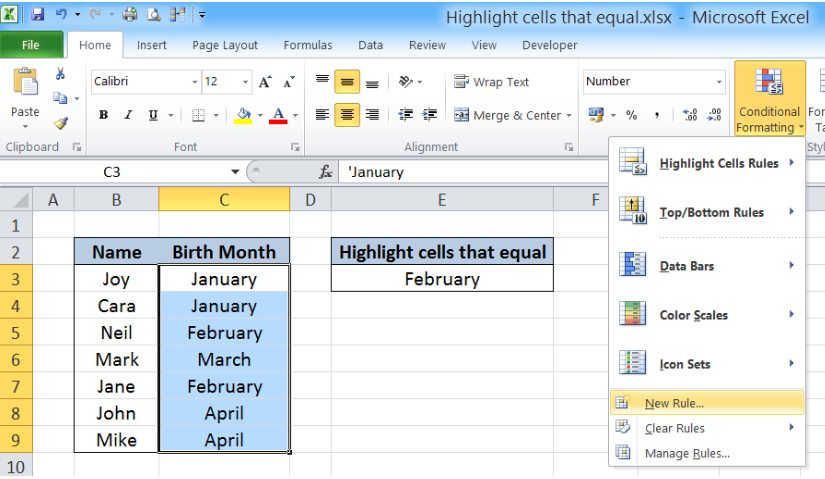Figure 3.  Creation of a new rule in conditional formatting

Step 3. Select the Rule Type “Use a formula to determine which cells to format” and enter this formula in the dialog box : =C3=\$E\$3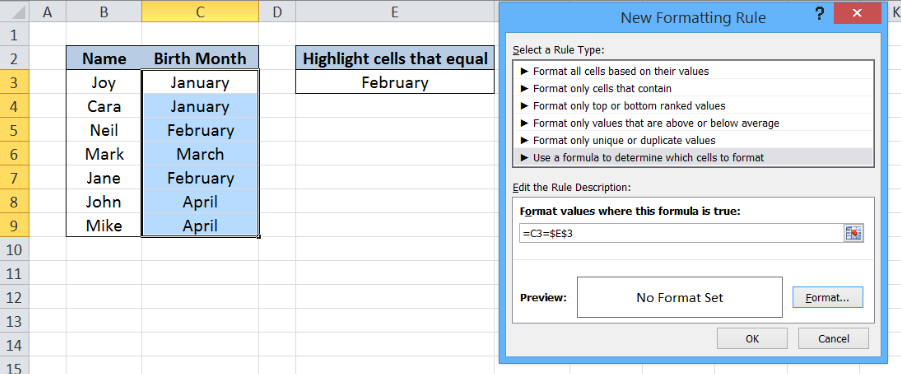Figure 4.  Entering the formula as a condition or formatting rule

Our formula =C3=\$E\$3 serves as the condition or rule that will trigger the conditional formatting.  For every cell in the range C3:C9, if the value is equal to the value in cell E3, which is “February”, the format will be changed.

The dollar sign “\$” fixes the cell E3 which is our basis for changing the format of the cells in column C.

To change the format, let us proceed to the next step.

Step 4. Click “Format” and then decide on the new format to apply to the cells in column C.  We can change the font, borders or fill the cells with different colors.

## Example

Select “Fill” and choose Orange, Accent 6, Lighter 60% and click OK.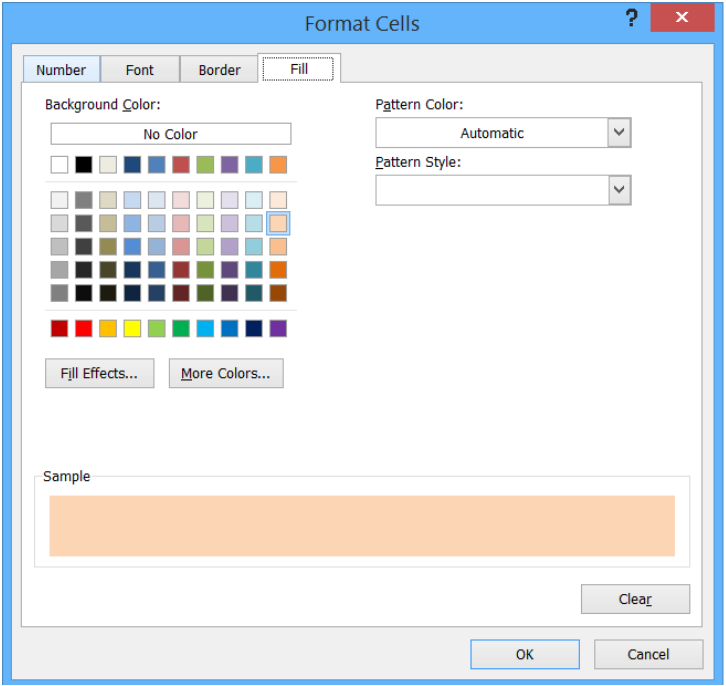Figure 5.  Selection of the format to use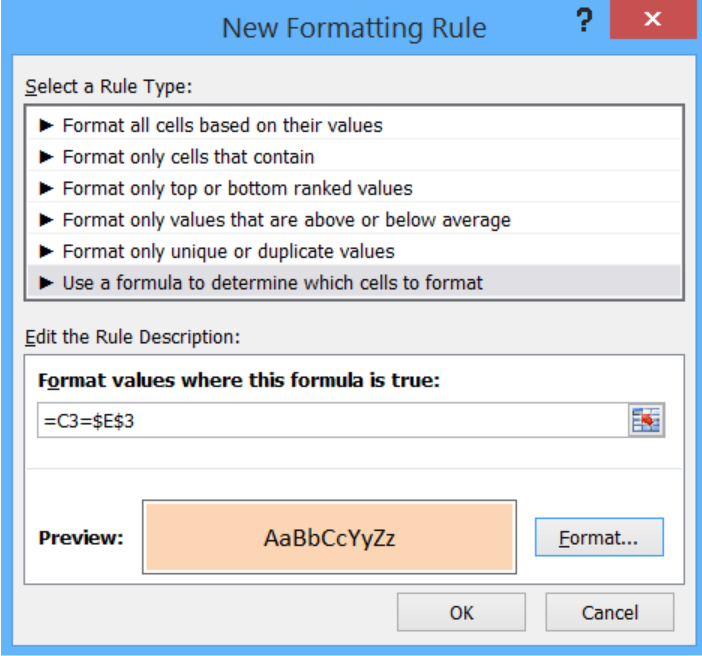Figure 6.  Completion of the new formatting rule with formula and format

This rule highlights the cells that satisfy the condition of C3=E3.  As a result, the cells that equal in value to E3 or “February” are highlighted as shown below.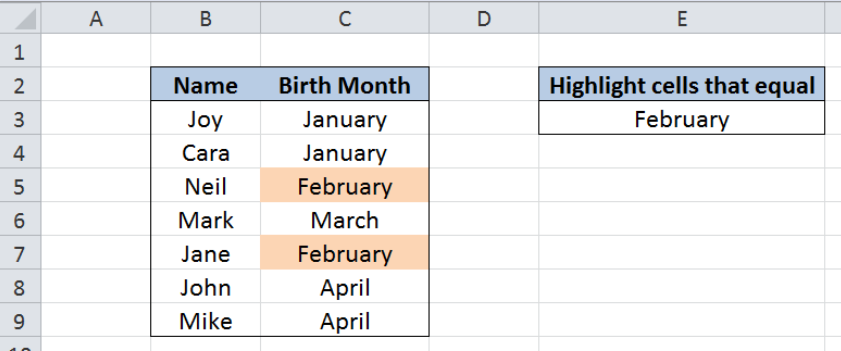Figure 7.  Output: New conditional formatting rule reflected in cells C5 and C7

With conditional formatting, we can now highlight the cells in column C that equal in value to cell E3.  Let us try changing the value in E3 to “march”.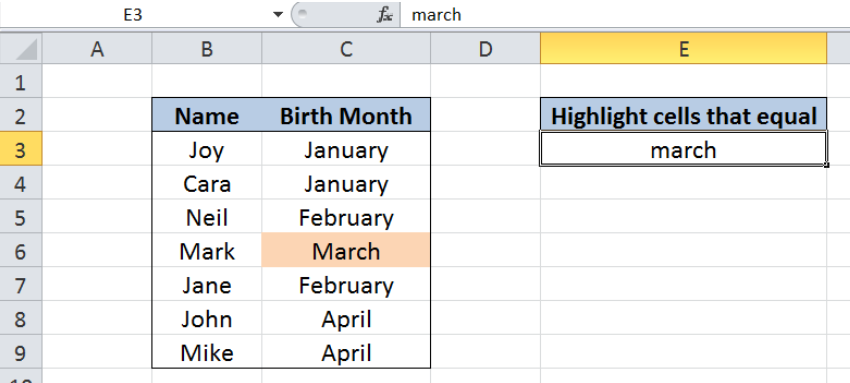Figure 8.  Highlighting cells that equal to “march”

The conditional formatting rule is automatically applied to cell C6 as shown above.  By just changing the value of one cell, we can now quickly highlight the specific cells in our worksheet with little effort.

## Notes

• The comparison of values is not case-sensitive, since the value in cell E3 is in lowercase while the highlighted value in C6 is in proper case.
• However, we can use the EXACT function to avoid case sensitivity errors. We can enter this formula instead: =EXACT(C3,\$E\$3)
• In highlighting cells that equal to a value, we can also enter that value directly into our formula in conditional formatting.  We can use this formula in our formatting rule:
=C3=”February”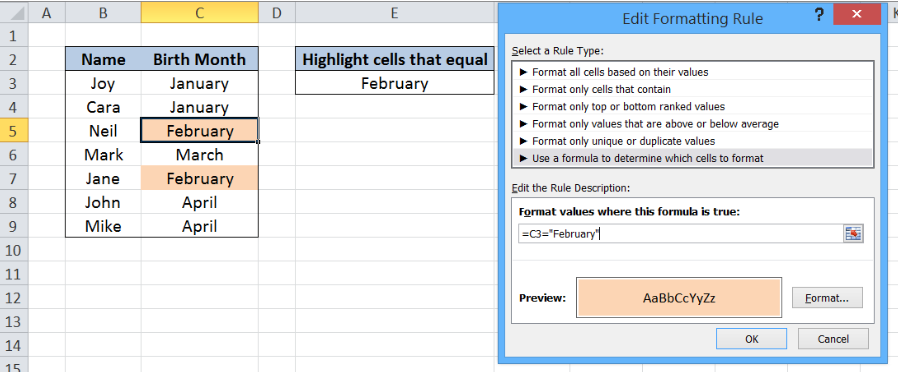Figure 9.  Output: Hardcoding the value “February” into the formatting rule

This method is longer and more tedious but it is also less prone to error.  As shown above, the result is the same as in the previous example where the formula we used was: =C3=\$E\$3.

Most of the time, the problem you will need to solve will be more complex than a simple application of a formula or function. If you want to save hours of research and frustration, try our live Excelchat service! Our Excel Experts are available 24/7 to answer any Excel question you may have. We guarantee a connection within 30 seconds and a customized solution within 20 minutes.

### Did this post not answer your question? Get a solution from connecting with the expert.Another blog reader asked this question today on Excelchat:
Solution examplesMy spreadsheet has several tabs. A formula on tab 1 is referencing data from tab 2. I want to duplicate my formula and then use the Replace function to have it reference tab 3 instead of tab 2. However, when I attempt the replace Excel opens a dialog box to open a different Excel file. Even if I forego the Replace function and just manually change the path within my formula, Excel opens the dialog box. At the moment I can't seem to reference any data on tab 3 on my spreadsheet. What is going on?
Solved by C. J. in 35 minsI need a formula to grab a value in column D on another spreadsheet where the corresponding value in the same row = a value found in row A on my spreadsheet
Solved by Z. F. in 15 minsHello I am trying to merge data are you able to help me? I have one column in a workbook that needs to be transfer to my master list
Solved by V. E. in 60 minsi want to write a formula like "if value of B2 exist in A:A show C2 value else show "NA"
Solved by V. A. in 20 minsI need to create a formula that fills finds the Sheet (col A) and then the Component (col B) and then adds the value I put in Col H into a cell in the corresponding cell of column of the found sheet.
Solved by Z. S. in 60 mins## Subscribe to Excelchat.coAnother blog reader asked this question today on Excelchat: